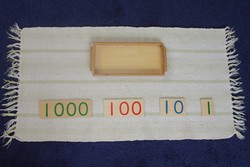# Introduction to the Cards

 What's new?!Our latest free printables are cards for the Logical Adjective Game and the Logical Adverb Game.
Introduction to the Cards
Math - Introduction to the Decimal SystemLevelPrimary
Age4+
PrerequisitesIntroduction to the Beads

This activity introduces the child to the numerals 1, 10, 100, and 1000.

## Presentation

1. Take the tray to a mat or table.
2. Lay out the cards as shown. The thousand should be on the left and the unit should be on the right.
3. Point to the 1, "This says 1."
4. Point to the 10, "This says 10."
5. Point to the 100, "This says 100. Can you count the zeros?"
6. Point to the 1000, "This says 1000. Can you count the zeros?"
7. Do a Three Period Lesson.

## Points of Interest

• Counting the zeros

## Material

### Where to Buy

see - Number Cards Material page